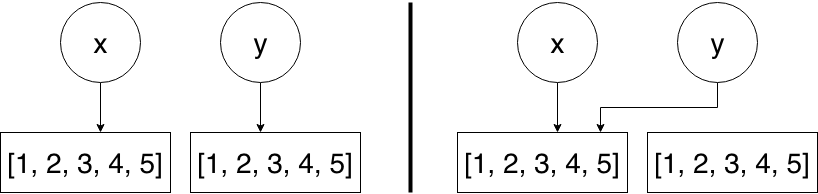# 4. Operators¶

In this section we’ll have a closer look at operators in Python. Operators are used to perform operations on variables and values. Python divides operators in the following groups:

• Arithmetic operators

• Assignment operators

• Comparison operators

• Logical operators

• Identity operators

• Membership operators

• Bitwise operators

We’ll have a look at all of them except bitwise operators as they are out of scope for this training session.

## 4.1. Arithmetic Operators¶

Arithmetic operators are used (as the name suggests) to perform arithmetical operations on numeric variables and values (e.g. `int` and `float`).

Note: Some of these arithmetic operators are implemented for more complex data structures like lists or dictionaries. However, they have a different meaning than the once discussed here.

Operator Name Example
- Subtraction x - y
* Multiplication x * y
/ Division x / y
% Modulus x % y
** Exponentiation x ** y
// Floor division x // y

The following code section shows you, how you could use the operators listed above.

```>>> x = 5
>>> y = 3
>>> x + 2
7
>>> x + y
8
>>> x * y
15
>>> 7 / 2  # always results in a float
3.5
>>> 7 // 2  # floor-division always results in an integer
3
>>> 7 % 2  # modulo operation -> returns the remainder of a division
1
>>> 7 ** 2  # square number of 7
49
```

## 4.2. Assignment Operators¶

Assignment operators are used to create or override variables. Besides the normal assignment operator `=`, which is used in the Variables section, there exist other assignment operators, which are a combination of the assignment operator and an arithmetical operator.

Operator Example Same As
= x = 5 x = 5
+= x += 3 x = x + 3
-= x -= 3 x = x - 3
*= x *= 3 x = x * 3
/= x /= 3 x = x / 3
%= x %= 3 x = x % 3
**= x **= 3 x = x ** 3
//= x //= 3 x = x // 3
```>>> x = 5
>>> x += 3
>>> x
8
>>> x %= 2
>>> x
0
```

## 4.3. Comparison Operators¶

Comparison operators are used to compare certain values (or variables) and get back a boolean value. Let’s assume we have two variables and want to know, whether they are equal or not:

```>>> x = 3
>>> y = 6
>>> x == y
False
>>> x != y
True
>>> x < y
True
```

Below you’ll find a list of available comparison operators.

Operator Name Example
== Equal x == y
!= Not equal x != y
> Greater than x > y
< Less than x < y
>= Greater than or equal to x >= y
<= Less than or equal to x <= y

## 4.4. Logical Operators¶

Logical operators are used to connect booleans logically to see, whether they are `True` or `False` within a certain context.

Operator Description Example
and Returns True if both statements are true x < 5 and x < 10
or Returns True if one of the statements is true x < 5 or x < 4
not Reverse the result, returns False if the result is true not(x < 5 and x < 10)
```>>> x = 3 == 3
>>> x
True
>>> y = 4 < 3
>>> y
False
>>> x and y
False
>>> x and not y
True
>>> x or y
True
```

## 4.5. Identity Operators¶

Identity operators are used to check the identity of an object.

Operator Description Example
is Returns true if both variables are the same object x is y
is not Returns true if both variables are not the same object x is not y

Note: Checking whether a value is `None` (equivalent to `null` in C or Java) is performed by using the identity operator `is` and not using the comparison operator `==`.

```>>> x = [1, 2, 3, 4, 5]
>>> y = [1, 2, 3, 4, 5]
>>> x is y
False
>>> x is not y
True
>>> y = x
>>> x is y
True
```

The following diagram illustrates, what happended in the example provided above.On the left-hand side you can see, that both variables point to independent lists. However, when we set `y = x`, `y` points to the same list as `x`, which you can see on the right-hand side.

## 4.6. Membership Operators¶

With membership operators you can check, whether a certain sequence is present in an object. For instance you could check if the number `5` is part of a list of grades or if the name Felix contains the letter `p`.

```>>> grades = [1, 2, 3, 4, 5, 6]
[1, 2, 3, 4, 5, 6]